Lakhmir Singh & Manjit Kaur: Force and Law of Motion, Solutions- 3

# Lakhmir Singh & Manjit Kaur: Force and Law of Motion, Solutions- 3 - Science Class 9

Page No - 75

Question 20:
Explain how a rocket works
Solution :
A rockets works on the principle of action and reaction. In a rocket, the hot gases produced by the rapid burning of fuel rush out of a jet at the bottom of the rocket at a very high speed. The equal and opposite reaction force of the downward going gases pushes the rocket upward with a great speed.

Question 21
Do action and reaction act on the same body or different bodies ? How are they related in magnitude and direction ? Are they simultaneous or not ?
Solution :
Action and reaction act on two different bodies. Action and reaction are equal in magnitude but they act in opposite directions and there is simultaneous action and reaction.

Question 22
If a man jumps out from a boat, the boat moves backwards. Why ?
Solution :
When a man jumps out from a boat, the boat moves backwards due to the fact that to step out of the boat, the man presses the boat with his foot in the backward direction. The push of the man on the boat is action. The boat exerts an equal force on the man in the forward direction and since the boat is not fixed and is floating, it moves backwards due to the action force exerted by the man.

Question 23
Why is it difficult to walk on a slippery road ?
Solution :
It becomes very difficult to walk on a slippery road because of the fact that on a slippery road, the friction is much less, and we cannot exert a backward action force on slippery ground which would produce a forward reaction force on us.

Question 24:
Explain why, a runner presses the ground with his feet before he starts his run.
Solution :
To start his run, a runner bends forward and pushes the ground with his feet in the backward direction. In turn ground exerts a reaction force on the runner in the forward direction which makes him run.

Question 25:
A 60 g bullet fired from a 5 kg gun leaves with a speed of 500 m/s. Find the speed (velocity) with which the gun recoils (jerks backwards).
Solution :
Mass of bullet, m1 = 60g = 0.06 kg
Velocity of bullet v1 = 500 m/s
Mass of gun m2 = 5 kg
Recoil velocity v2
According to the law of conservation of momentum
m1 x v1 = m2 x v2
0.06 x 500 = 5 x v2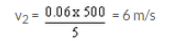Question 26:
A 10 g bullet travelling at 200 m/s strikes and remains embedded in a 2 kg target which is originally at rest but free to move. At what speed does the target move off ?
Solution :
Mass of bullet, m1 = 10g = 0.01 kg
Velocity of bullet v1 = 200 m/s
Mass of block with the bullet as bullet gets embedded in it, m2 = 2+0.01 = 2.01 kg
Recoil velocity v2
According to the law of conservation of momentum

m1 x v1 = m2 x v2
0.01 x 200 = 2.01 x v2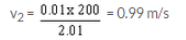Question 27:
A body of mass 2 kg is at rest. What should be the magnitude of force which will make the body move with a speed of 30 m/s at the end of 1 s ?
Solution :
Mass of the body = 2kg
Initial velocity u = 0
Final velocity v = 30 m/s
Time t = 1 s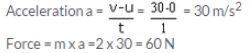Question 28:
A body of mass 5 kg is moving with a velocity of 10 m/s. A force is applied to it so that in 25 seconds, it attains a velocity of 35 m/s. Calculate the value of the force applied.
Solution :
Mass of the body = 5 kg
Initial velocity u = 10 m/s
Final velocity v = 35 m/s
Time t = 25 s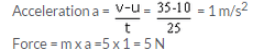Question 29:
A car of mass 2400 kg moving with a velocity of 20 m s-1 is stopped in 10 seconds on applying brakes. Calculate the retardation and the retarding force.
Solution :
Mass of the car = 2400 kg
Initial velocity u = 20 m/s
Final velocity v = 0 m/s
Time t = 10 s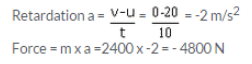Question 30:
For how long should a force of 100 N act on a body of 20 kg so that it acquires a velocity of 100 m/s ?
Solution :
Mass of the body = 20 kg
Initial velocity u = 0 m/s
Final velocity v = 100 m/s
Force F = 100 N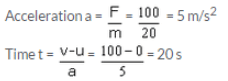Question 31:
How long will it take a force of 10 N to stop a mass of 2.5 kg which is moving at 20 m/s ?
Solution :
Mass of the body = 2.5 kg
Initial velocity u = 20 m/s
Final velocity v = 0 m/s
Force F = 10 N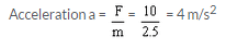since v<u, so acceleration will have a negative sign a = -4m/s2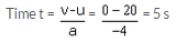Question 32:
The velocity of a body of mass 10 kg increases from 4 m/s to 8 m/s when a force acts on it for 2 s.
(a) What is the momentum before the force acts ?
(b) What is the momentum after the force acts ?
(c) What is the gain in momentum per second ?
(d) What is the value of the force ?
Solution :
Mass of the body = 10 kg
Initial velocity u = 4 m/s
Final velocity v = 8 m/s
Time t = 2 s
a) Momentum before force acts p1= m x u = 10 x 4 = 40 kg.m/s
b) Momentum after force acts p2 = m x v = 10 x 8 = 80 kg.m/s
c) Gain in momentum for 2 s = p2-p1 =40 kg.m/sQuestion 33:
A gun of mass 3 kg fires a bullet of mass 30 g. The bullet takes 0.003 s to move through the barrel of the gun and acquires a velocity of 100 m/s. Calculate :
(i) the velocity with which the gun recoils.
(ii) the force exerted on gunman due to recoil of the gun
Solution :
Mass of the gun m1 = 3 kg
Mass of bullet m2 = 30 g = 0.03 kg
Velocity of bullet v2 =100 m/s
i) According to the law of conservation of momentum
m1 x v1 = m2 x v2
3 x v1= 0.03 x 100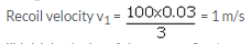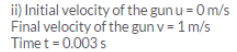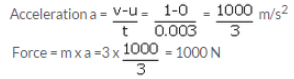Question 34:
Draw a diagram to show how a rocket engine provides a force to move the rocket upwards. Label the diagram appropriately.
Solution :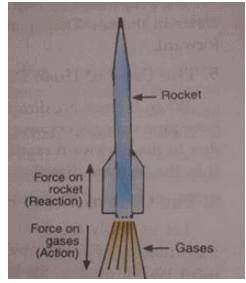Question 35:
Name the laws involved in the following situations :
(a) the sum of products of masses and velocities of two moving bodies before and after their collision remains the same.
(b) a body of mass 5 kg can be accelerated more easily by a force than another body of mass 50 kg under similar conditions
(c) when person A standing on roller skates pushes another person B (also standing on roller skates) and makes him move to the right side, then the person A himself gets moved to the left side by an equal distance.
(d) if there were no friction and no air resistance, then a moving bicycle would go on moving for ever.

Solution :
a)Law of conservation of momentum
b)Newton’s second law of motion
c) Newton’s third law of motion
d)Newton’s first law of motion

Question 36:
(a) State and explain Newton’s second law of motion.
(b) A 1000 kg vehicle moving with a speed of 20 m/s is brought to rest in a distance of 50 metres :
(i) Find the acceleration.
(ii) Calculate the unbalanced force acting on the vehicle.

Solution :
(a) According to Newton’s second law of motion: The rate of change of momentum of a body is directly proportional to the applied force, and takes place in the direction in which the force acts.
Consider a body of mass m having initial velocity u. the initial momentum of this body will be mu. Suppose a force F acts on this body for time t causing the final velocity to be v. The final momentum of the body will be mv. Now the change in momentum is mv < mu and the time taken for this change is t. So according to Newton’s second law of motion,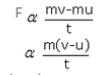but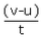, represents changes in velocity with time i.e acceleration 'a'. so by replacing  with a in the above relation, we get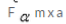thus the force acting on a body is directly proportional to the product of mass and acceleration produced in the body by the action of the force.thus newton second law gives the relationship between force and aceeleration.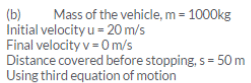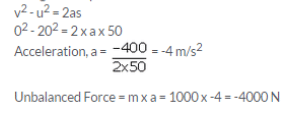Question 37:
(a) Explain why, a cricket player moves his hands backwards while catching a fast cricket ball.
(b) A 150 g ball, travelling at 30 m/s, strikes the palm of a player’s hand and is stopped in 0.05 second. Find the force exerted by the ball on the hand.
Solution :
a) A player moves his hands backwards while catching a fast ball because a fast moving ball has a large momentum and in stopping this ball, its momentum has
to be reduced to zero. Now, when a cricket player moves back his hands on catching the fast ball, then the time taken to reduce the momentum of the ball is increased. So, the rate of change of momentum of ball is decreased and hence a small force is exerted on the hands of the player and the hands of the player do not get hurt.
b)Mass of ball = 150 g = 0.15 kg
Initial velocity u = 30 m/s
Final velocity v = 0 m/s
Time t = 0.05 s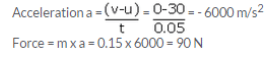Question 38:
(a) State Newton’s third law of motion and give two examples to illustrate the law.
(b) Explain why, when a fireman directs a powerful stream of water on a fire from a hose pipe, the hosepipe tends to go backward.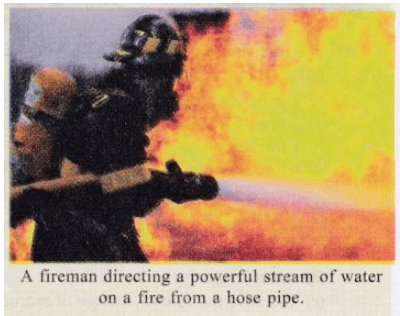Solution :
a) According to Newton’s third law of motion: Whenever one body exerts a force on another body, the second body exerts an equal and opposite force on the first body. In other words, to every action, there is an equal and opposite reaction. Two examples to illustrate this law-
When a man jumps out from a boat, the boat moves backward. This is due to the fact that to step out of the boat, the man presses the boat with his foot in the backward direction. The push of the man on the boat in action. The boat exerts an equal force in the forward direction and since the boat is not fixed and is floating, it moves backward due to the action force exerted by the man.
Gunman gets a jerk on firing a bullet from his gun. This is because when a bullet is fired from a gun, the force sending the bullet forward is equal to the force sending the gun backwards but due to high mass of the gun, it moves only a little distance backward giving a jerk to the gunman.
b) When a fireman directs a powerful stream of water on a fire, the hosepipe tends to go backward due to the reaction force of the water rushing through it in the forward direction at a great speed.

Page No - 76

Question 39:
(a) State the law of conservation of momentum.
(b) Discuss the conservation of momentum in each of the following cases :
(i) a rocket taking off from ground.
(ii) flying of a jet aeroplane.
Solution :
a) According to the law of conservation of momentum: When two (or more) bodies act upon one another, their total momentum remains constant (or conserved) provided no external forces are acting. It means that when one body gains momentum, then some other body loses an equal amount of momentum i.e. momentum is neither created nor destroyed.
b)
a. Rocket taking off from the ground The chemicals inside the rocket burn and produce very high velocity blast of hot gases. These gases pass out through the tail nozzle of the rocket in the downward direction with tremendous speed and the rocket moves up to balance the momentum of the gases. The gases have a very high velocity ang hence a very large momentum. An equal momentum is imparted to the rocket in the opposite direction, so that it goes up with a high velocity.
b. Flying of jet aeroplane
In jet aeroplanes, a large volume of gases produced by the combustion of fuel is allowed to escape through a jet in backward direction. Due to high velocity, the backward rushing gases have a large momentum. They impart an equal and opposite momentum to the jet aeroplane due to which it moves forward with a great speed.

Question 40:
(a) If a balloon filled with air and its mouth untied/ is released with its mouth in the downward direction, it
moves upwards. Why ?
(b) An unloaded truck weighing 2000 kg has a maximum acceleration of 0.5 m/s2. What is the maximum acceleration when it is carrying a load of 2000 kg ?
Solution :
a) If of a balloon filled with compressed air and its mouth untied is released with its mouth in the downward direction, the balloon moves in the upward direction because the air present in the balloon rushes out in the downward direction. The equal and opposite reaction of downward going air pushes the balloon upwards.
b) Mass of the unloaded truck, m1= 2000 kg
Acceleration a1 = 0.5 m/s2
Mass of loaded truck, m2 = 2000+ 2000 = 4000 kg
Acceleration a2
m1 x a1 = m2 x a2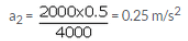Page No:77

Question 51:
Why are car seat-belts designed to stretch some what in a collision ?
Solution :
Car seat-belts are somewhat stretchable so as to increase the time taken by the passengers to fall forward. Due to this, the rate of change of momentum of passengers is reduced and hence less stopping force acts on them. So the passengers do not get hurt.

Question 52:
The troops (soldiers) equipped to be dropped by parachutes from an aircraft are called paratroopers. Why do paratroopers roll on landing ?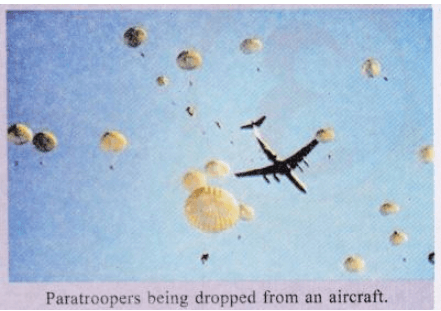Solution :
The paratroopers roll on landing to increase the time taken to reduce the momentum of their body. Thus, the rate of change of momentum is reduced and hence less force is exerted on their legs and they do not get hurt.

Question 53:
Why would an aircraft be unable to fly on the moon ?
Solution :
An aircraft needs air because air moving under the wings of aircraft is strong enough to hold it up and air is also required to burn the fuel in aircraft engines. Since there is no air on moon, an aircraft cannot fly on moon.

Question 54:
Explain why it is possible for a small animal to fall from a considerable height without any injury being caused when it reaches the ground.
Solution :
It is possible for a small animal to fall from a considerable height without being injured because a small animal has small mass, so the momentum produced is less. When the small animal falls to the ground with less momentum, less opposing force of ground acts on it and hence no injury is caused to it

Question 55:
A boy of mass 50 kg running at 5 m/s jumps on to a 20 kg trolley travelling in the same direction at 1.5 m/s. What is their common velocity ?
Solution :
Mass of the boy, m1= 50 kg
Speed of boy, u1 = 5 m/s
Mass of trolley m2 = 20 kg
Speed of trolley u2 =1.5 m/s
Combined mass of boy and trolley, m = 20+ 50 = 70 kg
Combined velocity v
Acc. to the law of conservation of momentum
m1u1 + m2u2 = mv
50 x 5 + 20 x 1.5 = 70 x v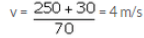Question 56:
A girl of mass 50 kg jumps out of a rowing boat of mass 300 kg on to the bank, with a horizontal velocity of 3 m/s. With what velocity does the boat begin to move backwards ?
Solution :
Mass of the boat mb = 300 kg
Velocity of boat vb
Mass of girl mg = 50 kg
Velocity of girl vg = 3 m/s
Acc. to the law of conservation of momentum
mbvb=mgvg
300 x vb = 50 x 3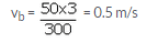Question 57:
A truck of mass 500 kg moving at 4 m/s collides with another truck of mass 1500 kg moving in the same direction at 2 m/s. What is their common velocity just after the collision if they move off together ?
Solution :
Mass of first truck, m1= 500 kg
Speed of first truck, u1 = 4 m/s
Mass of second truck, m2 = 1500 kg
Speed of second truck, u2 =2 m/s
Combined mass of both trucks, m = 1500 + 500 = 2000 kg
Combined velocity v
Acc. to the law of conservation of momentum
m1u1 + m2u2 = mv
500 x 4 + 1500 x 2 = 2000 x v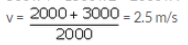Question 58:
A ball X of mass 1 kg travelling at 2 m/s has a head-on collision with an identical ball Y at rest. X stops and Y moves off. Calculate the velocity of Y after the collision.
Solution :
Mass of the ball x, m1= 1 kg
Speed of ball x, u1 = 2 m/s
Mass of ball y, m2 = 1 kg
Speed of ball y, u2 =0 m/s (at rest)
Velocity of ball x after collision, v1 = 0 m/s
Velocity of ball y after collision, v2
Acc. to the law of conservation of momentum
m1u1 + m2u2 = m1v1 + m2v2
1 x 2 + 1 x 0 = 1 x 0 + 1 x v2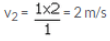Question 59:
A heavy car A of mass 2000 kg travelling at 10 m/s has a head-on collision with a sports car B of mass 500 kg. If both cars stop dead on colliding, what was the velocity of car B ?
Solution :
Mass of car A, m1= 2000 kg
Speed of car A, v1 = 10 m/s
Mass of car B, m2 = 500 kg
Speed of car B, v2
Acc to law of conservation of momentum
m1v1 = m2v2
2000 x 10 = 500 x v2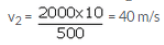Question 60:
A man wearing a bullet-proof vest stands still on roller skates. The total mass is 80 kg. A bullet of mass 20 grams is fired at 400 m/s. It is stopped by the vest and falls to the ground. What is then the velocity of the man ?
Solution :
Mass of the man, m1= 80 kg
Speed of man, v1
Mass of bullet m2 = 20 g = 0.02 kg
Speed of bullet v= 400 m/s
Acc to law of conservation of momentum
m1v1 = m2v2
80 x v1 = 0.02 x 400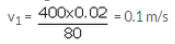The document Lakhmir Singh & Manjit Kaur: Force and Law of Motion, Solutions- 3 | Science Class 9 is a part of the Class 9 Course Science Class 9.
All you need of Class 9 at this link: Class 9

## FAQs on Lakhmir Singh & Manjit Kaur: Force and Law of Motion, Solutions- 3 - Science Class 9

 1. What are the three laws of motion?Ans. The three laws of motion, as stated by Sir Isaac Newton, are: 1. Newton's First Law of Motion: An object at rest will stay at rest, and an object in motion will stay in motion with the same speed and direction, unless acted upon by an external force. 2. Newton's Second Law of Motion: The acceleration of an object is directly proportional to the net force acting on it and inversely proportional to its mass. It can be mathematically represented as F = ma, where F is the force, m is the mass, and a is the acceleration. 3. Newton's Third Law of Motion: For every action, there is an equal and opposite reaction. This means that whenever an object exerts a force on another object, the second object exerts an equal and opposite force on the first object.
 2. What is inertia?Ans. Inertia is the property of an object to resist any change in its state of motion. According to Newton's First Law of Motion, an object at rest tends to stay at rest, and an object in motion tends to stay in motion with the same speed and direction unless acted upon by an external force. Inertia is directly related to mass, where objects with greater mass have more inertia and are harder to accelerate or decelerate.
 3. How does force affect the motion of an object?Ans. Force is the external influence that can change the state of motion or shape of an object. According to Newton's Second Law of Motion, the acceleration of an object is directly proportional to the net force acting on it and inversely proportional to its mass. This means that a larger force applied to an object will result in a greater acceleration, while a smaller force will result in a smaller acceleration. Force can also change the direction of motion or bring the object to rest.
 4. What is the difference between mass and weight?Ans. Mass and weight are two different physical quantities. Mass is a measure of the amount of matter in an object and is a scalar quantity, while weight is the force exerted on an object due to gravity and is a vector quantity. Mass is constant and does not change with location, while weight varies with the strength of gravity. Mass is measured in kilograms (kg), while weight is measured in newtons (N).
 5. Can an object be in motion without any external force acting on it?Ans. According to Newton's First Law of Motion, an object at rest will stay at rest, and an object in motion will stay in motion with the same speed and direction, unless acted upon by an external force. This means that an object can be in motion without any external force acting on it if there are no other forces present to change its state of motion or bring it to rest. However, in reality, it is difficult to find a completely isolated system where no external forces are present.

## Science Class 9

98 videos|364 docs|148 tests

## Science Class 9

98 videos|364 docs|148 tests
Signup to see your scores go up within 7 days! Learn & Practice with 1000+ FREE Notes, Videos & Tests.
10M+ students study on EduRev
Track your progress, build streaks, highlight & save important lessons and more!(Scan QR code)
Related Searches

,

,

,

,

,

,

,

,

,

,

,

,

,

,

,

,

,

,

,

,

,

,

,

,

;